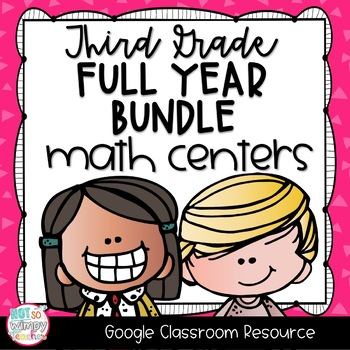3rd
Subjects
Standards
Resource Type
Formats Included
•Google Drive™ folder
Pages
230 pages
\$40.00
Bundle
List Price:
\$49.50
You Save:
\$9.50
\$40.00
Bundle
List Price:
\$49.50
You Save:
\$9.50This bundle contains one or more resources with Google apps (e.g. docs, slides, etc.).

### Description

Did your students love to do math centers in class, but now you're needing to teach virtually? These digital math centers will have your students practicing and reviewing their math skills AND having fun while doing it.

This is a money saving bundle! If you purchased the sets individually, it would cost \$50! Buying the bundle means that you are getting almost two whole sets for FREE!

With movable parts that students can click and drag, students will be so engaged in the activities they won't even realize that they are learning!

These digital math centers are created on Google slides.

They are a perfect solution for at-home math practice and review if your students are participating in remote learning. They are also perfect as an independent math center to use in class on devices. Additionally, these centers can be used for morning work.

Each center includes a slide with simple, student-friendly directions. This allows students to easily navigate each center independently. There are also tips along the way that show students how to use different technology tools, which are used to help them solve a variety of math problems.

There are ten different sets for a total of 93 different centers. That's enough for the entire school year!!!

Sets included:

1. Back to School

2. Place Value

4. Multiplication

5. Division

6. Fractions

7. Time and Elapsed Time

8. Measurement and Data

9. Fractions

10. Geometry (Freebie!)

The resource includes:

• detailed teacher directions for assigning and grading the digital centers in Google Classroom
• student-friendly directions
• 93 interactive center activities on Google Slides

*********

I also have this resource in a printable version! Click HERE to check it out.

Total Pages
230 pages
Included
Teaching Duration
N/A
Report this Resource to TpT
Reported resources will be reviewed by our team. Report this resource to let us know if this resource violates TpT’s content guidelines.

### Standards

to see state-specific standards (only available in the US).
Interpret products of whole numbers, e.g., interpret 5 × 7 as the total number of objects in 5 groups of 7 objects each. For example, describe a context in which a total number of objects can be expressed as 5 × 7.
Interpret whole-number quotients of whole numbers, e.g., interpret 56 ÷ 8 as the number of objects in each share when 56 objects are partitioned equally into 8 shares, or as a number of shares when 56 objects are partitioned into equal shares of 8 objects each. For example, describe a context in which a number of shares or a number of groups can be expressed as 56 ÷ 8.
Use multiplication and division within 100 to solve word problems in situations involving equal groups, arrays, and measurement quantities, e.g., by using drawings and equations with a symbol for the unknown number to represent the problem.
Determine the unknown whole number in a multiplication or division equation relating three whole numbers. For example, determine the unknown number that makes the equation true in each of the equations 8 × ? = 48, 5 = __ ÷ 3, 6 × 6 = ?.
Apply properties of operations as strategies to multiply and divide. Examples: If 6 × 4 = 24 is known, then 4 × 6 = 24 is also known. (Commutative property of multiplication.) 3 × 5 × 2 can be found by 3 × 5 = 15, then 15 × 2 = 30, or by 5 × 2 = 10, then 3 × 10 = 30. (Associative property of multiplication.) Knowing that 8 × 5 = 40 and 8 × 2 = 16, one can find 8 × 7 as 8 × (5 + 2) = (8 × 5) + (8 × 2) = 40 + 16 = 56. (Distributive property.)